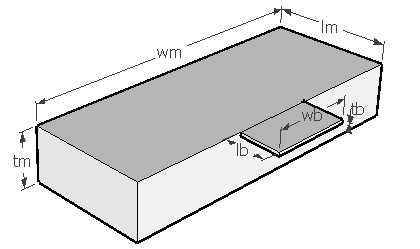MASFREQ,lb,wb,tb,lm,wm,tm,den,ymod,sel
Vibration frequency of a cantilever beam with an end mass

lb          length of the cantilever in µm
wb         width of the cantilever in µm
tb          thickness of the cantilever in µm
lm         length of the mass in µm
wm        width of the mass in µm
tm         thickness of the mass in µm
den       density of the material in kg/m3
ymod    Young's modulus of the material of the beam in GPa
sel         number denoting the selected result.
Use 2 for first harmonic frequencyNotes

A mass suspended from a cantilever is used in MEMS devices for sensing inertial signals like in accelerometers. This design interface can be used to estimate the first harmonic frequency of the system. The displacement is assumed to be perpendicular to the plane of the cantilever. For mathematical modeling, the beam-mass system is considered as a compound cantilever in a fixed-free configuration. The limitation in this analysis is that the mass is usually rigid and will not be subjected to bending as experienced by the beam.

The plot shows the amplitude frequency relationship for the given beam-mass system. It shows the first resonance frequency as a sharp rise in amplitude. Using the cross hair tool, the resonant frequency and the corresponding relative amplitude can be obtained.

Assumptions

-The default material is Silicon with a Young's modulus of 180GPa and Poisson's ratio of 0.3.
-The beam and mass has uniform cross section.
-The weight of the mass and beam are uniformly distributed.
-The property of the material remain constant across the beam-mass structure.
-Damping is not considered.
-Axial loading and cross-axis bending are not considered.